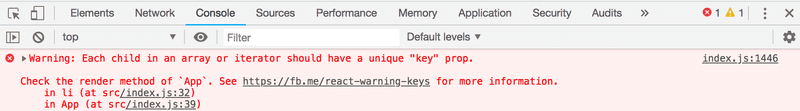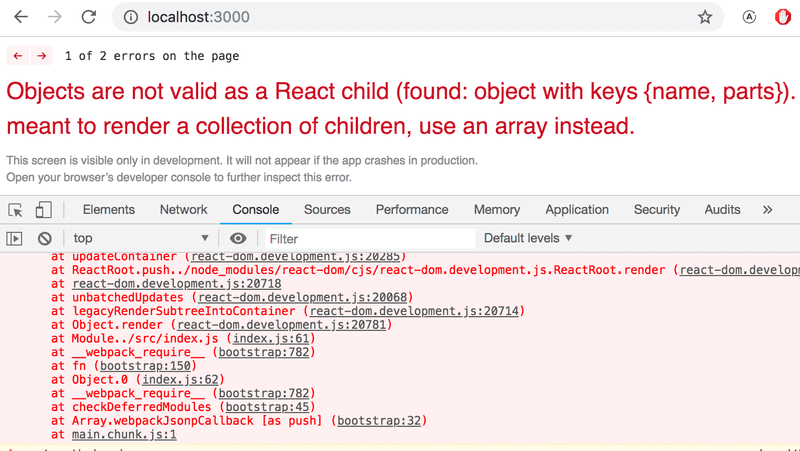a

# 从渲染集合到模块学习

### console.log

What's the difference between an experienced JavaScript programmer and a rookie? The experienced one uses console.log 10-100 times more.

``console.log('props value is' + props)``

``console.log('props value is', props)``

``props value is [Object object]``

### Protip: Visual Studio Code snippets

【高级技巧: Visual Studio Code 的代码片段】

``````{
"console.log": {
"prefix": "clog",
"body": [
"console.log('\$1')",
],
"description": "Log output to console"
}
}``````

【JavaScript 数组】

【事件处理复习】

### Rendering Collections

【渲染集合】

• Note 应用实际上是在创建一个和提醒、便笺相关的应用，因此以下的Note均翻译为便笺。

``````import React from 'react'

const App = (props) => {
const { notes } = props

return (
<div>
<h1>Notes</h1>
<ul>
<li>{notes.content}</li>
<li>{notes.content}</li>
<li>{notes.content}</li>
</ul>
</div>
)
}

export default App``````

index.js 内容如下:

``````import ReactDOM from 'react-dom'
import App from './App.js'

const notes = [
{
id: 1,
content: 'HTML is easy',
date: '2019-05-30T17:30:31.098Z',
important: true
},
{
id: 2,
content: 'Browser can execute only JavaScript',
date: '2019-05-30T18:39:34.091Z',
important: false
},
{
id: 3,
content: 'GET and POST are the most important methods of HTTP protocol',
date: '2019-05-30T19:20:14.298Z',
important: true
}
]

ReactDOM.render(
<App notes={notes} />,
document.getElementById('root')
)``````

``<li>{notes.content}</li>``

``notes.map(note => <li>{note.content}</li>)``

``````[
<li>HTML is easy</li>,
<li>Browser can execute only JavaScript</li>,
<li>GET and POST are the most important methods of HTTP protocol</li>,
]``````

``````const App = (props) => {
const { notes } = props

return (
<div>
<h1>Notes</h1>
<ul>        {notes.map(note => <li>{note.content}</li>)}      </ul>    </div>
)
}``````

``````const App = (props) => {
const { notes } = props

return (
<div>
<h1>Notes</h1>
<ul>
{notes.map(note =>
<li>            {note.content}          </li>        )}
</ul>
</div>
)
}``````

### Key-attribute

【Key-属性】``````const App = (props) => {
const { notes } = props

return (
<div>
<h1>Notes</h1>
<ul>
{notes.map(note =>
<li key={note.id}>            {note.content}          </li>        )}
</ul>
</div>
)
}``````

React 使用数组中对象的key属性来确定组件在重新渲染时，如何更新组件生成的视图。 更多的说明在React 文档

### Map

``````const notes = [
{
id: 1,
content: 'HTML is easy',
date: '2019-05-30T17:30:31.098Z',
important: true
},
{
id: 2,
content: 'Browser can execute only JavaScript',
date: '2019-05-30T18:39:34.091Z',
important: false
},
{
id: 3,
content: 'GET and POST are the most important methods of HTTP protocol',
date: '2019-05-30T19:20:14.298Z',
important: true
}
]``````

``````const result = notes.map(note => note.id)
console.log(result)``````

map 总是会创建一个新数组，其元素是从原始数组的元素通过mapping映射创建的，映射的逻辑是使用作为 map 方法传递进去的函数。

``note => note.id``

``````(note) => {
return note.id
}``````

``const result = notes.map(note => note.content)``

``````notes.map(note =>
<li key={note.id}>{note.content}</li>
)``````

``note => <li key={note.id}>{note.content}</li>``

### Anti-pattern: Array Indexes as Keys

【反模式: 将数组的索引作为键】

``notes.map((note, i) => ...)``

``````<ul>
{notes.map((note, i) =>
<li key={i}>
{note.content}
</li>
)}
</ul>``````

### Refactoring modules

【重构模块】

``````const App = ({ notes }) => {  return (
<div>
<h1>Notes</h1>
<ul>
{notes.map(note =>
<li key={note.id}>
{note.content}
</li>
)}
</ul>
</div>
)
}``````

``````const Note = ({ note }) => {  return (    <li>{note.content}</li>  )}
const App = ({ notes }) => {
return (
<div>
<h1>Notes</h1>
<ul>
{notes.map(note =>           <Note key={note.id} note={note} />        )}      </ul>
</div>
)
}``````

``````import ReactDOM from 'react-dom'
import App from './App.js'``````

Note.js 文件的内容如下:

``````import React from 'react'

const Note = ({ note }) => {
return (
<li>{note.content}</li>
)
}

export default Note``````

``````import React from 'react'
import Note from './components/Note'
const App = ({ notes }) => {
// ...
}``````

``'./components/Note'``### When the Application Breaks

【当应用挂掉了】``````const Course = ({ course }) => (
<div>
</div>
)

const App = () => {
const course = {
// ...
}

return (
<div>
<Course course={course} />
</div>
)
}``````

``````const App = () => {
const course = {
// ...
}

console.log('App works...')
return (
// ..
)
}````````````const Course = ({ course }) => (
<div>
</div>
)``````

``````const Course = ({ course }) => {
console.log(course)  return (
<div>
</div>
)
}``````

``````const Course = (props) => {  console.log(props)  const { course } = props
return (
<div>